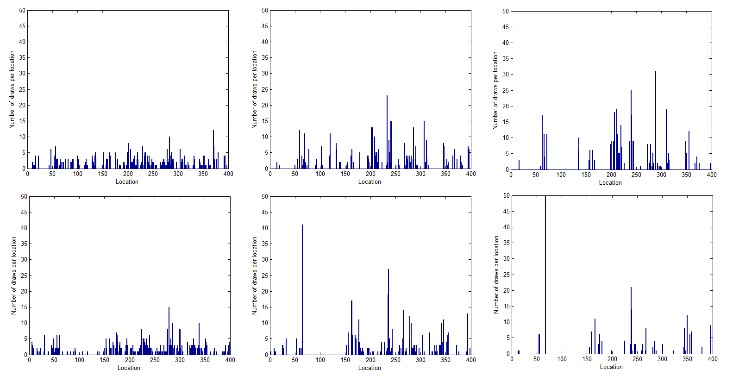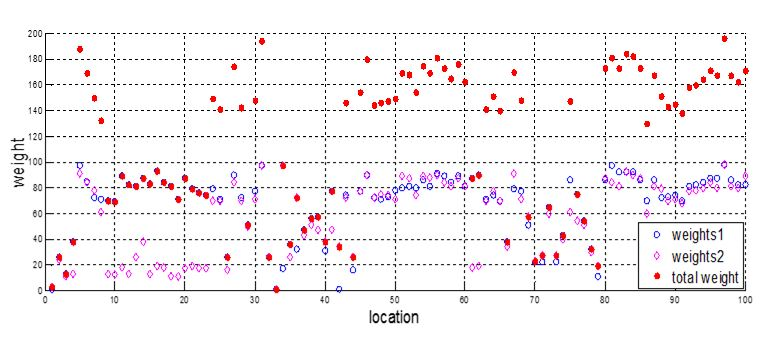# Studying uncertainty for robot localization with multiple sensors using Monte Carlo Localization

Mobile robots have gained a lot of attention in the last years with a lot of applications in various fields. The robot’s ability to navigate its environment of operation is critical in fulfilling its mission. One of the key tasks in robot navigation is the self-localization which is simply determining the position of the robot in the environment. With the increased complexity of the environment, the level of uncertainty in determining the robot’s location increases and MCL (Monte Carlo Localization) is an efficient method in such cases.

The MCL algorithm is a probabilistic approach that considers the locations in the environment and assigns a certain weight for how likely the robot is at each location. To evaluate such likelihood, the algorithm needs the map for the environment and some sensor(s) measurements for the features in the environment as well as a motion model.

We are interested in exploring the weights distribution in case of only 1 type of sensors and comparing it to using 2 types. To experiment this, we used the LEGO mindstorms NXT kit with the light and ultrasonic sensors. With the given map, readings from the sensors are fed to the algorithm for determining the location and a comparison between the two cases is made. We used histograms to visualize the probability distributions and here we consider specifically the case of symmetric environment.

In such a case, the robot is confused as where the true location might be. However, if we make use of another feature in the environment we can get better results as shown in the figure below. The upper row describes the weights distribution using one sensor and the lower one using two sensors. Clearly many locations have high equal weights in upper row while in the lower one, the algorithm could converge to the true location with relatively high confidence.Another way to look into this is seeing the weights distribution using one sensor or a combination of two in the symmetric environment. The below figure illustrates this. The way the final weight is computed is as follows. For every set of weights a threshold is computed statistically to set a rule for which weights are high and which weights are low. The final weights to be considered for resampling is calculated as the sum of the two weights if both are above the thresholds and the maximum of them otherwise. The value of threshold needs to be selected carefully because it may create negative results or may end up being like having only one sensor. We chose it as a fraction of the maximum weight, which could be enhanced over multiple iterations (time steps).Contact: Hassan Shehawy: hassan.shehawi@ut.ee